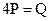Chapter 5, Problem 35P### Principles of Economics 2e

2nd Edition
Steven A. Greenlaw; David Shapiro
ISBN: 9781947172364

#### Solutions

Chapter
Section### Principles of Economics 2e

2nd Edition
Steven A. Greenlaw; David Shapiro
ISBN: 9781947172364
Textbook Problem

# The equation for a supply curve isWhat is the elasticity of supply as price rises from 3 to 4? What is the elasticity of supply as the price rises from 7 to 8? Would you expect these answers to be the same?

To determine

Calculate the elasticity of supply for given supply function when price rises from 3 to 4 and 7 to 8. Also, mention would we expect the same answer in both cases.

4P = Q.

Explanation

We will first find the quantity supplied at price $3 4P = Q4×3= Q Q = 12Q = 12 units at P =$3

Now, we will find quantity supplied at price $4 4P = Q4×4 = QQ =16Q = 16 units at P =$4

Now, we can calculate the elasticity of supply with the following formula:

e = ΔQΔP×PQ

e = 161243×312e = 41×14e = 1

Therefore, the elasticity of supply is 1 when price rises from $3 to$4.

Now we will calculate the elasticity of supply when price rises from $7 to$8

### Still sussing out bartleby?

Check out a sample textbook solution.

See a sample solution

#### The Solution to Your Study Problems

Bartleby provides explanations to thousands of textbook problems written by our experts, many with advanced degrees!

Get Started